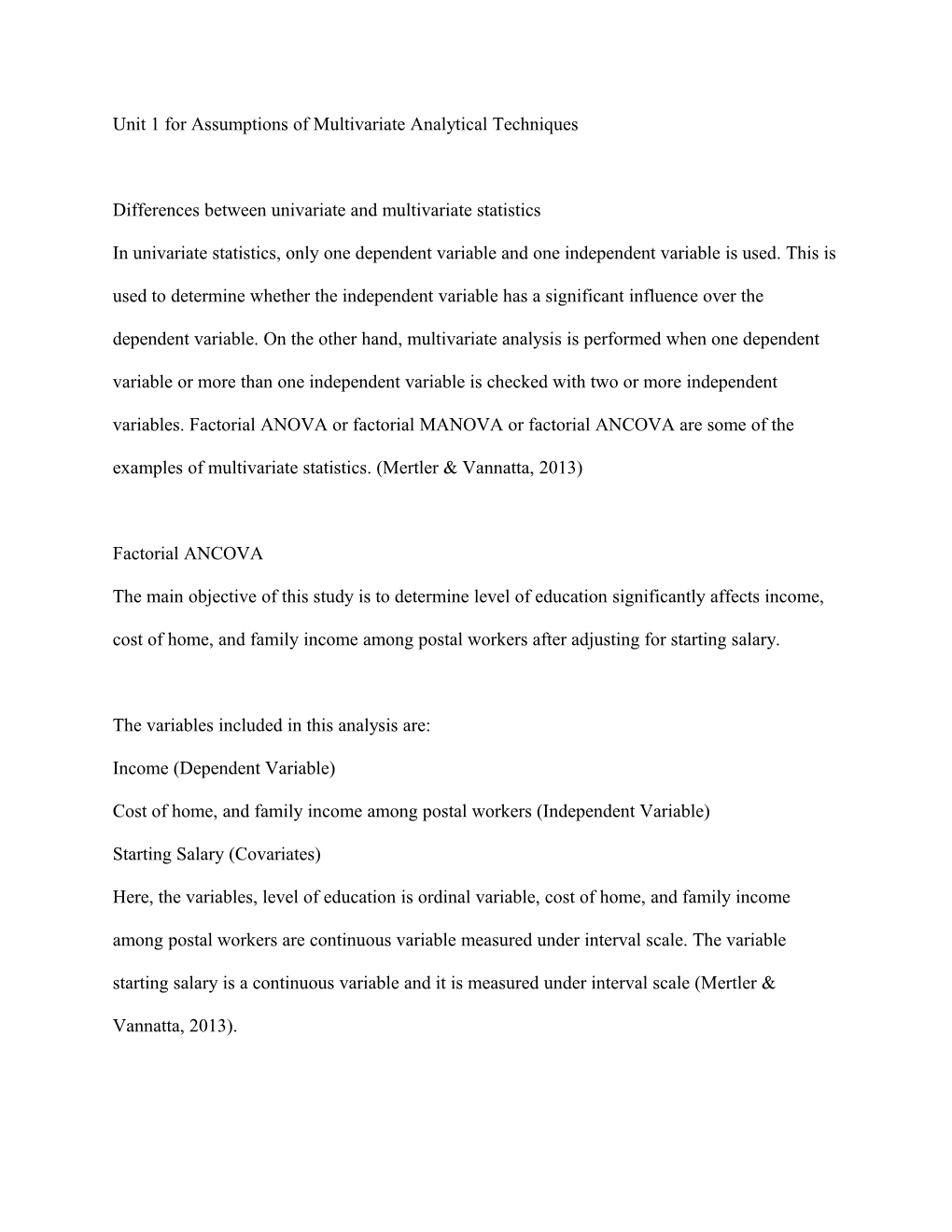# Unit 1 for Assumptions of Multivariate Analytical TechniquesUnit 1 for Assumptions of Multivariate Analytical Techniques

Differences between univariate and multivariate statistics

In univariate statistics, only one dependent variable and one independent variable is used. This is used to determine whether the independent variable has a significant influence over the dependent variable. On the other hand, multivariate analysis is performed when one dependent variable or more than one independent variable is checked with two or more independent variables. Factorial ANOVA or factorial MANOVA or factorial ANCOVA are some of the examples of multivariate statistics. (MertlerVannatta, 2013)

Factorial ANCOVA

The main objective of this study is to determine level of education significantly affects income, cost of home, and family income among postal workers after adjusting for starting salary.

The variables included in this analysis are:

Income (Dependent Variable)

Cost of home, and family income among postal workers (Independent Variable)

Starting Salary (Covariates)

Here, the variables, level of education is ordinal variable, cost of home, and family income among postal workers are continuous variable measured under interval scale. The variable starting salary is a continuous variable and it is measured under interval scale (MertlerVannatta, 2013).

In order to test the normality assumption Shapiro Wilk test for normality is used and if p – value of the Shapiro Wilk test is greater than 0.05, then we conclude that the assumption of normality is satisfied. (Field, 2013)

For this study, we use factorial ANCOVA to test the claim that level of education significantly affects income, cost of home, and family income among postal workers after adjusting for starting salary.

The Analysis of Covariance (ANCOVA) is a type of Analysis of Variance (ANOVA) that is used to control for potential confounding variables. Logistic regression adjusting for confounding variables is also used to control for potential confounding variables.

Decision Making Tool

The perfect decision making tool to perform the factorial ANCOVA is SPSS. Since this is a user friendly tool, this tool does not require coding to generate the output and also it is easy to access. The other statistical tools which perform factorial ANCOVA are SAS, R, Minitab, Mathlab and Stata.

References

Field, A. (2013). Discovering statistics using IBM SPSS Statistics (4th ed.). Thousand Oaks, CA: Sage.

Mertler, C. A., & Vannatta, R. A. (2013). Advanced and multivariate statistical methods (5th ed.). Glendale, CA: Pyrczak Publishing.